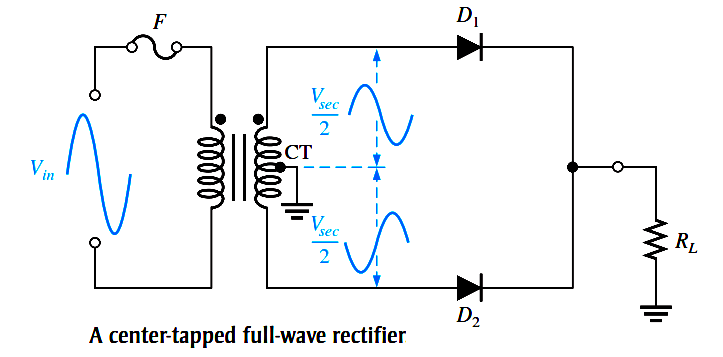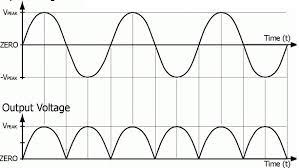### Full Wave Rectifier

Center Tap Full Wave Rectifier

A rectifier circuit whose transformer  secondary is tapped  to get the desired  output voltage, using two diodes alternatively,  to rectify the complete cycle is called a Center-tapped Full wave rectifier circuit. The transformer is center tapped here unlike the other cases.1. In center  tap rectifier output voltage frequency is twice the input voltage frequency.

2. In this input  is passed in both cycle ie in positive and negative cycle.

Parameter of Full Wave Rectifier:

• Average output voltage: Average value/dc value/mean value= Area over one period /Total time period =2Vm/

• Average output current = 2Vm/𝝅R

• RMS output voltage: It is root mean square value of output voltage = Vm/√2

• RMS output current: It is root mean square value of current = Vm/√2R

• RMS value of AC component: Iac(rms) =0.385*Im

• Input power: Pin = Irms*Irms*R

• Output power: Pout =Idc*Idc*R

• Efficiency: 𝜼 = Pout/Pin=0.81

• PIV: IT is maximum voltage across diode in reverse bias condition. PIV=2Vm

• Ripple factor: 𝜸=unwanted comp /wanted comp

=  Iac(rms)/Idc

=0.482